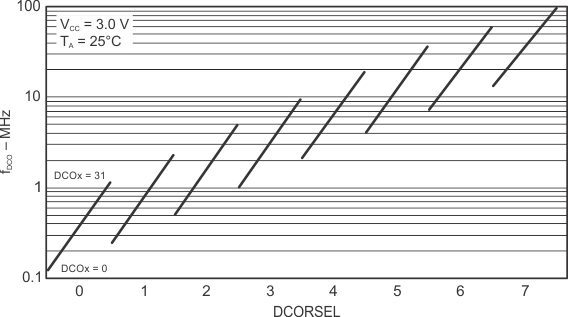SLAS619R August   2010  – September 2018

PRODUCTION DATA.

• YFF|40
• RSB|40
• DA|38
• RSB|40
• DA|38

### 5.25 DCO Frequency

over recommended ranges of supply voltage and operating free-air temperature (unless otherwise noted) (see Figure 5-22)
PARAMETER TEST CONDITIONS MIN TYP MAX UNIT
fDCO(0,0) DCO frequency (0, 0)(1) DCORSELx = 0, DCOx = 0, MODx = 0 0.07 0.20 MHz
fDCO(0,31) DCO frequency (0, 31)(1) DCORSELx = 0, DCOx = 31, MODx = 0 0.70 1.70 MHz
fDCO(1,0) DCO frequency (1, 0)(1) DCORSELx = 1, DCOx = 0, MODx = 0 0.15 0.38 MHz
fDCO(1,31) DCO frequency (1, 31)(1) DCORSELx = 1, DCOx = 31, MODx = 0 1.47 3.45 MHz
fDCO(2,0) DCO frequency (2, 0)(1) DCORSELx = 2, DCOx = 0, MODx = 0 0.32 0.75 MHz
fDCO(2,31) DCO frequency (2, 31)(1) DCORSELx = 2, DCOx = 31, MODx = 0 3.17 7.38 MHz
fDCO(3,0) DCO frequency (3, 0)(1) DCORSELx = 3, DCOx = 0, MODx = 0 0.64 1.51 MHz
fDCO(3,31) DCO frequency (3, 31)(1) DCORSELx = 3, DCOx = 31, MODx = 0 6.07 14.0 MHz
fDCO(4,0) DCO frequency (4, 0)(1) DCORSELx = 4, DCOx = 0, MODx = 0 1.3 3.2 MHz
fDCO(4,31) DCO frequency (4, 31)(1) DCORSELx = 4, DCOx = 31, MODx = 0 12.3 28.2 MHz
fDCO(5,0) DCO frequency (5, 0)(1) DCORSELx = 5, DCOx = 0, MODx = 0 2.5 6.0 MHz
fDCO(5,31) DCO frequency (5, 31)(1) DCORSELx = 5, DCOx = 31, MODx = 0 23.7 54.1 MHz
fDCO(6,0) DCO frequency (6, 0)(1) DCORSELx = 6, DCOx = 0, MODx = 0 4.6 10.7 MHz
fDCO(6,31) DCO frequency (6, 31)(1) DCORSELx = 6, DCOx = 31, MODx = 0 39.0 88.0 MHz
fDCO(7,0) DCO frequency (7, 0)(1) DCORSELx = 7, DCOx = 0, MODx = 0 8.5 19.6 MHz
fDCO(7,31) DCO frequency (7, 31)(1) DCORSELx = 7, DCOx = 31, MODx = 0 60 135 MHz
SDCORSEL Frequency step between range DCORSEL and DCORSEL + 1 SRSEL = fDCO(DCORSEL+1,DCO)/fDCO(DCORSEL,DCO) 1.2 2.4 ratio
SDCO Frequency step between tap DCO and DCO + 1 SDCO = fDCO(DCORSEL,DCO+1)/fDCO(DCORSEL,DCO) 1.02 1.12 ratio
Duty cycle Measured at SMCLK 40% 50% 60%
dfDCO/dT DCO frequency temperature drift fDCO = 1 MHz, VCORE = 1.2 V, 2.0 V 0.1 %/°C
dfDCO/dVCORE DCO frequency voltage drift fDCO = 1 MHz 1.9 %/V
When selecting the proper DCO frequency range (DCORSELx), the target DCO frequency, fDCO, should be set to reside within the range of fDCO(n, 0),MAX ≤ fDCO ≤ fDCO(n, 31),MIN, where fDCO(n, 0),MAX represents the maximum frequency specified for the DCO frequency, range n, tap 0 (DCOx = 0) and fDCO(n,31),MIN represents the minimum frequency specified for the DCO frequency, range n, tap 31 (DCOx = 31). This ensures that the target DCO frequency resides within the range selected. It should also be noted that if the actual fDCO frequency for the selected range causes the FLL or the application to select tap 0 or 31, the DCO fault flag is set to report that the selected range is at its minimum or maximum tap setting.Figure 5-22 Typical DCO Frequency